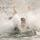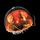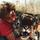## Social Question# Brain Teaser: How well do you understand weighted averages?

Asked by LostInParadise (28574) December 9th, 2009

You have used weighted averages if you have ever tried to compute your grade in a class where regular tests and finals were weighed differently. Weighted averages show up in a wide variety of applications. Weighted averages for two quantities have some particularly nice properties.

The following problem came up in an online tutoring session. I solved it with the student using some messy algebra, because I suspected the student did not understand weighted averages. If you use weighted averages, this particular problem is set up so it can be solved fairly easily without any algebra. Do you see how to do it? Also let me know if you were ever taught in school about weighed averages.

How much of a 2% solution of sulfuric acid must be used to dilute 5 liters of a 10% solution to get a 7% solution?

Observing members: 0Composing members: 0I think most people do understand weighted averages, they just don’t know that they do. Is there any difference between the problem you wrote and this problem?:

If 5 students score 10 points on a quiz, the rest of the class got 2 points, and the class average is 7 points, how many students got a score of 2?

If stated like that, the problem is less scary. Come to think of it, I’m not sure this is a weighted average, to me it’s just a normal mathematical averaging problem. Then again, I’ve never understood exactly what a weighted average is.

hannahsugs (3238)“Great Answer” (1) Flag as…Why messy algebra versus real (simple) algebra? Indeed, as @hannahsugs stated, this isn’t really a weighted average, since there really is no average here.

((0.02 * x) + (0.10 * 5))/(x+5) = 0.07

becomes

0.02x + 0.5 = 0.07x + 0.35

becomes

0.15 = 0.05x

becomes

x = 0.15/0.05 = 3

x= 3 liters

shilolo (18045)“Great Answer” (4) Flag as…syz (35649)“Great Answer” (0) Flag as…3 liters is the correct answer.

@shilolo , That is precisely the messy algebra I was talking about.

@svz It is a particular interest of mine because weighted averages show up in a lot of different applications

And this is a weighted average problem.
You want to find a weighted average of 10% and 2% to arrive at 7%.

The problem is equivalent to having a 5 pound weight at a distance of 10 and trying to find what weight to place at a distance of 2 to get the center of gravity to be at a distance of 7. If you know how to balance with levers, the solution is fairly easy.

LostInParadise (28574)“Great Answer” (0) Flag as…It might be semantics, but I view this as straightforward algebra. Calling it a weighted average makes it sound different, but as I illustrated above, it’s fairly simple to compute. Solving a weighted averages such as a final grade in a class where attendance = 10%, homework = 30% and tests = 60% knowing all the scores is an obvious weighted average. Once you switch it to solving for x, it’s plainly an algebra problem.

shilolo (18045)“Great Answer” (0) Flag as…Finding a center of gravity is an example of use of weighted averages. I recast the problem in terms of distances being weighted by real weights. How much more of a weighted average problem could there be?

The point is that the form of the equations is identical in each instance, even though the physical circumstances are different. In each case you have an equation of the form:
sum of (wi * xi)/ (sum of wi). The xi, wi can be test scores and numbers of students or acid concentrations and volumes or distances and weights.

LostInParadise (28574)“Great Answer” (0) Flag as…What difference does it make? Trust me, I’ve taken many advanced math classes. This is a straightforward as it comes.

shilolo (18045)“Great Answer” (0) Flag as…Finding a center of gravity is an example of use of weighted averages. I recast the problem in terms of distances being weighted by real weights. How much more of a weighted average problem could there be?

The point is that the form of the equations is identical in each instance, even though the physical circumstances are different. In each case you have one quantity being weighted by another. The equations are of the form:
sum of (wi * xi)/ (sum of wi). The xi, wi can be test scores and numbers of students or acid concentrations and volumes or distances and weights.

LostInParadise (28574)“Great Answer” (0) Flag as…Using the lever analogy, you get 5 pounds at a distance of (10 -7)=3. The distance corresponding to the 2% solution is (7–2)=5. For a lever, balance is achieved when distance times weight is the same on both ends. To get 5*3 in both cases, the weight would have to be 3. That is, 3 liters of 2% solution. And not an x in sight.

LostInParadise (28574)“Great Answer” (0) Flag as…You are simply recasting all of your algebra problems and calling them “weighted averages”, but at their core, they are algebra problems. Definitions of weighted mean here, here, here and here all present the weighted mean as I defined it. If thinking about algebra this way helps you, so be it, as long as you are getting the right answers.

shilolo (18045)“Great Answer” (0) Flag as…The definition you provide is exactly the same as the one I provided. I am not casting all algebra problems as weighted average ones, only those where the definition applies.

The weighted average approach is simpler and more elegant because it breaks the problem down into two very straightforward ones – computing the ratio of volumes and then using that ratio to find the missing volume. Not only is the weighted average approach computationally simpler, but it provides an insight not otherwise apparent, namely that in working with solutions of different concentrations to achieve a target concentration, the ratio of volumes of the two solutions used will always be the same. In this case, using a 10% solutions and a 2% solution to create a 7% solution will always require volumes of the two solutions in a 5:3 ratio, regardless of what else is specified.

LostInParadise (28574)“Great Answer” (0) Flag as…I see I did a horrible job of explaining this. It is true that the algebra inovolved in either case is not that extensive, but seeing things from the weighted average point of view simplifies things. So here follows what I should have said.

If you have a lever with two weights w1 and w2 with respective distances d1 and d2 from the balance point then the law of the lever says that w1*d1=w2*d2. This can easily be derived from the fact that the balance point is the center of gravity and the result generalized for any weighted average.

Let d1 and d2 be given with respect to some arbitrary origin and let d3 be the coordinate of the balance point.. Then we have:
w1*d1 + w2*d2 = (w1+w2)*d3.
A little algebraic manipulation gives:
w1(d1-d3)=w2(d3-d2), which is essentially the law of the lever.

This result holds for any weighted average and is intuitive and easy to remember. When dealing with volumes and concentrations we have that in general, combining two volumes v1 and v2 with concentrations of c1 and c2 combined to give concentration c3:
v1*c1 + v2*c2 = (v1+v2)*c3, which is a weighted average

In the given problem, v1=5, v2 is to be determined, c1=.1, c2=.02 and c3=.07.
Applying the law of the lever, we can write:
5(.1 = .07) = (.07 – .02)x, and the problem is immediately solved.

LostInParadise (28574)“Great Answer” (0) Flag as…